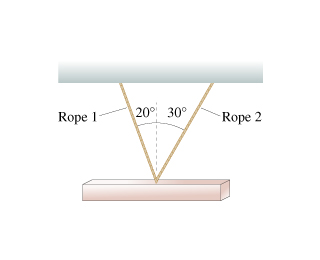# A 1100 kg steel beam is supported by two ropes. What is the tension in each?

edited

A 1100 kg steel beam is supported by two ropes. What is the tension in each?

Figure:by
selected by

T1*sin 20 = T2*sin 30

T2 = T1*sin 20/sin 30 = T1*0.6840

1100*g = T1*cos20+0,684T1*cos30

1100*9.8 = T1(cos20+0.684cos 30) = 1.532*T1

T1 = 1100*9.8/1.532 = 7035 N

T2 = T1*0.684 = 4812 N

check = 7035*0.940+4812*0.866 = 10780 N

1100*9.8 = 10780 N

Lorem ipsum dolor sit amet, consectetur adipiscing elit, sed do eiusmod tempor incididunt ut labore et dolore magna aliqua. Ut enim ad minim veniam, quis nostrud exercitation ullamco laboris nisi ut aliquip ex ea commodo consequat. Duis aute irure dolor in reprehenderit in voluptate velit esse cillum dolore eu fugiat.
by

Weight = 1100 * 9.8 = 10,780 N

The sum of the vertical components of the two tension forces is equal to the weight of the beam. The horizontal components of the two tension forces are equal in magnitude.

T1 * cos 20 + T2 * cos 30 = 10,780

T1 * sin 20 = T2 * sin 30

T1 = T2 * 2 * sin 20

T2 * 2 * sin 20 * cos 20 + T2 * cos 30 = 10,780

T2 = 10,780 ÷ (2 * sin 20 * cos 20 + cos 30)

This is approximately 7144.7 N.

T1 = [10,780 ÷ (2 * sin 20 * cos 20 + cos 30)] * 2 * sin 20

This is approximately 4,887 N.

Lorem ipsum dolor sit amet, consectetur adipiscing elit, sed do eiusmod tempor incididunt ut labore et dolore magna aliqua. Ut enim ad minim veniam, quis nostrud exercitation ullamco laboris nisi ut aliquip ex ea commodo consequat. Duis aute irure dolor in reprehenderit in voluptate velit esse cillum dolore eu fugiat.
by

Can't get the pic right now, but assuming the ropes are equally offset from middle of the beam and that the beam has uniform section:

1100*9.81 = 2*T

Lorem ipsum dolor sit amet, consectetur adipiscing elit, sed do eiusmod tempor incididunt ut labore et dolore magna aliqua. Ut enim ad minim veniam, quis nostrud exercitation ullamco laboris nisi ut aliquip ex ea commodo consequat. Duis aute irure dolor in reprehenderit in voluptate velit esse cillum dolore eu fugiat.